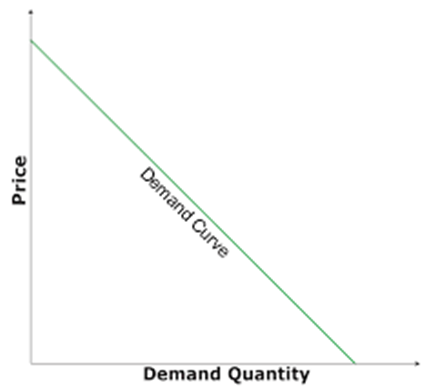Economics (NCERT) Notes

2.15 Relationship between Elasticity and Expenditure

Elasticity and Expenditure•The expenditure on a good is equal to the (demand for the good)x(price)
•The price of a good and the demand for the good are inversely related to each other.
•Whether the expenditure on the good goes up or down as a result of an increase in its price depends on how responsive the demand for the good is to the price change.

Effect of Increase in Price
•If the percentage decline in quantity is greater than the percentage increase in the price, the expenditure on the good will go down.
•If the percentage decline in quantity is less than the percentage increase in the price, the expenditure on the good will go up
•If the percentage decline in quantity is equal to the percentage increase in the price, the expenditure on the good will remain unchanged

Effect of decline in the price
•If the percentage increase in quantity is greater than the percentage decline in the price, the expenditure on the good will go up
•If the percentage increase in quantity is less than the percentage decline in the price, the expenditure on the good will go
•If the percentage increase in quantity is equal to the percentage decline in the price, the expenditure on the good will remain unchanged

Expenditure and Elasticity
•The expenditure on the good would change in the opposite direction as the price change if and only if the percentage change in quantity is greater than the percentage change in price, i.e. if the good is price-elastic.
•The expenditure on the good would change in the same direction as the price change if and only if the percentage change in quantity is less than the percentage change in price, i.e., if the good is price inelastic
•The expenditure on the good would remain unchanged if and only if the percentage change in quantity is equal to the percentage change in price, i.e., if the good is unit-elastic

Relationship between Elasticity and Expenditure
•Suppose
•at price p, the demand for a good is q, and
•at price p + ∆ p, the demand for the good is q + ∆ q.
•Expenditure
•At price p, the total expenditure on the good is pq, and
•At price p + ∆ p, the total expenditure on the good is (p + ∆ p)(q + ∆ q).
•If price changes from p to (p + ∆ p),
•the change in the expenditure on the good (∆ E )
= (p + ∆ p)(q + ∆ q) – pq
= qp + pq + ∆ pq
=qp + pq (For small values of ∆ p and ∆ q)
= p q[1 +p/q(q/ ∆ p)]
=p q[1 +eD)]

Relationship between Elasticity and Expenditure
•If price changes from p to (p + ∆ p), the change in the expenditure on the good ∆ E =p q[1 +eD)]
•if eD < –1 (elastic), then q (1 + eD) < 0, and hence, ∆ E has the opposite sign as ∆ p,
•if eD > –1 (inelastic), then q (1 + eD) > 0, and hence, ∆ E has the same sign as ∆ p,
•if eD = 1 (unit-elastic), then q (1 + eD) = 0, and hence, ∆ E = 0.

 Related Articles
 • 6.8 Behaviour of Firms in Oligopoly • 6.1 Non-competitive Markets • 5.8 Applications of Supply-Demand Analysis • 5.6 Market Equilibrium: Free Entry and Exit • 5.5 Impact of Shift in Supply and Demand • 5.2 Market Equilibrium for Fixed Number of Firms • 5.1 Market Equilibrium • 4.10 Price Elasticity of Supply • 4.9 Market supply Curve • 8.1 Importance of Infrastructure
 Recent Articles
 • Q9. A journalist fighting the stone mafia • Innovation and Creativity • Love and hatred • Religion and Spirituality • Tulsidas • Bureaucrat at the Temple • Getting Fooled for Kindness • Burning the boat • Three Masons • Two Salesmen